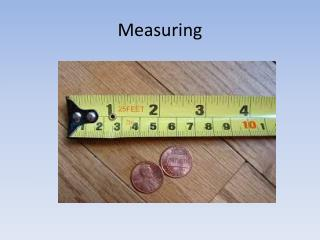DownloadDownload PresentationMeasuring

# Measuring

Télécharger la présentation## Measuring

- - - - - - - - - - - - - - - - - - - - - - - - - - - E N D - - - - - - - - - - - - - - - - - - - - - - - - - - -
##### Presentation Transcript

1. Measuring

2. Accuracy v. Precision pg. 8 Precision - how closely the measurements match each other. Instruments that measure using more decimals are more precise. Accuracy – how closely the measurement matches the true or accepted value.

3. Accurate Precise

4. Accurate • Precise

5. Accurate • Precise

6. Accurate • Precise

7. Accurate • Precise

8. How To Read Scientific Instruments • 1) Record the _______ digit on the instrument. • 2) Then _______________ the last digit.

9. How to Count Significant Figures Count all of the digits you are sure of. Add the last digit you estimate.

10. Percent Error • When quantities have accepted or true values, we can calculate the percent error between our measurement of the value and the accepted value with the formula

11. How to Read a Graduated Cylinder • Sit the graduated cylinder on a level surface. • Lower your eyes to the level of the liquid (called the meniscus). • Take your reading from the bottom of the meniscus. • Count all of the digits you are sure of. • Add the last digit that you estimate.

12. Quiz • Number your paper 1 to 15.

13. 1) Measuring Length 2 cm 19 cm 1.95 cm 1.95238 cm

14. 2) Measuring Length • 10cm • 1.1cm • 1.05cm • 1.526cm

15. 3) Measuring Length • 17 cm • 17.5 cm • 18.0 cm • 17.5082 cm

16. 4) Measuring Length • 4cm • 4.3cm • 4.30cm • 4.3057cm

17. 5) Measuring Length • 12 cm • 12.0 cm • 12.7 cm • 12.0953 cm

18. 6) Measuring Liquids • The meniscus is the curve in the upper surface of a liquid close to the surface of the container or another object, caused by surface tension. It can be either convex or concave. Is this meniscus convex or concave? 6) _________________ For questions 7 to 12, measure the amount of liquid in each graduated cylinder by reading the bottom of the meniscus.

19. 7) Measuring Liquid Volume • 10ml • 10.3ml • 11.5ml • 13.0ml

20. 8) Measuring Liquid Volume • 266 ml • 287 ml • 290 ml • 294 ml

21. 9) Measuring Liquid Volume • 52 ml • 53 ml • 54.5 ml • 52.8 ml

22. 10) Measuring Liquid Volume • 80 ml • 80.0 ml • 81.0 ml • 79.0 ml

23. 11) Measuring Liquid Volume • 2.8 ml • 2.80 ml • 2.90 ml • 2.9 ml

24. 12) Measuring Liquid Volume • 4.90 ml • 4.80 ml • 5.0 ml • 5.0853 ml

25. 13) Measuring Solids • 625.62g • 26.57g • 265.73 • 526.6g

26. 14) Measuring Solids • 373.33g • 734.33g • 374.33g • 373.3g

27. 15) Measuring Solids • 222.50g • 221.50g • 221.5g • 221.0g